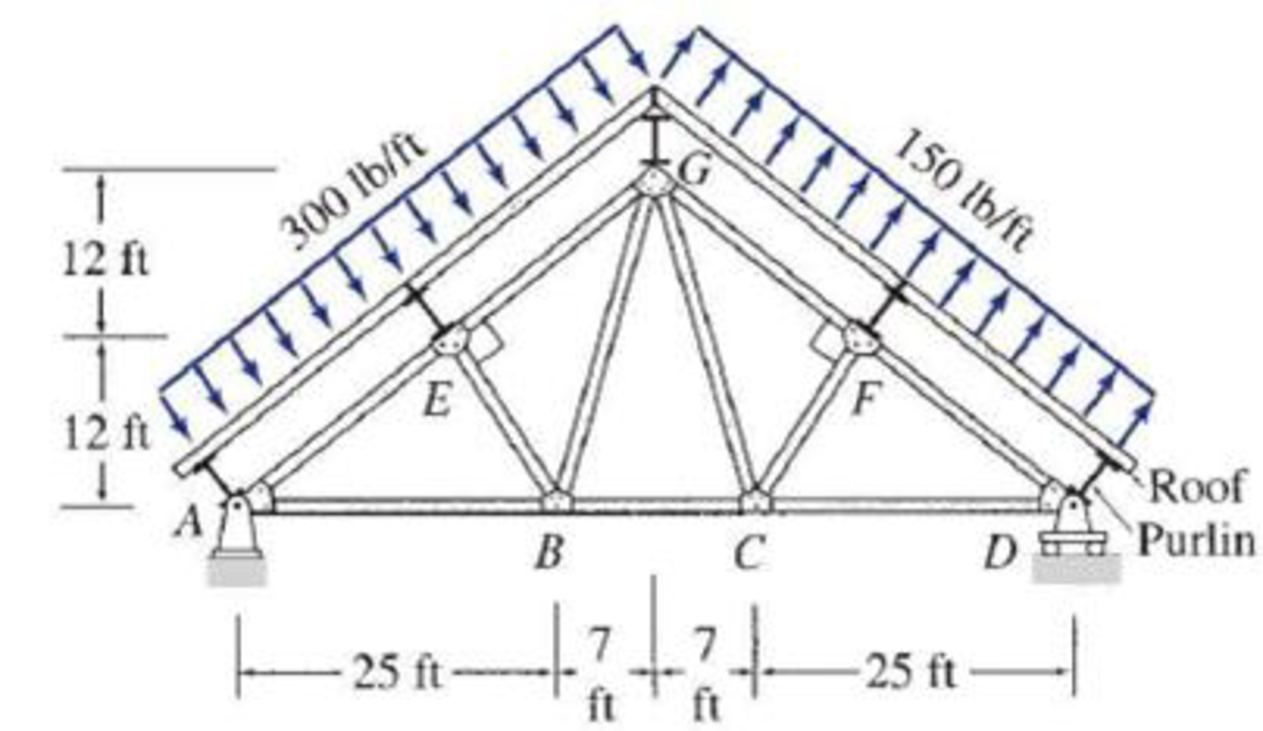# 4.30 and 4.31 Determine the force in each member of the roof truss shown. The roof is simply supported on purlins which, in turn, are attached to the joints of the top chord of the truss. Thus, the uniformly distributed loading on the roof is transmitted by the purlins as concentrated loads to the truss joints. FIG. P4.30

#### Solutions

Chapter
Section
Chapter 4, Problem 30P
Textbook Problem
1544 views

## 4.30 and 4.31 Determine the force in each member of the roof truss shown. The roof is simply supported on purlins which, in turn, are attached to the joints of the top chord of the truss. Thus, the uniformly distributed loading on the roof is transmitted by the purlins as concentrated loads to the truss joints.FIG. P4.30

To determine

Find the forces in the members of the roof truss.

### Explanation of Solution

Given information:

Apply the sign conventions for calculating reactions, forces and moments using the three equations of equilibrium as shown below.

• For summation of forces along x-direction is equal to zero (Fx=0), consider the forces acting towards right side as positive (+) and the forces acting towards left side as negative ().
• For summation of forces along y-direction is equal to zero (Fy=0), consider the upward force as positive (+) and the downward force as negative ().
• For summation of moment about a point is equal to zero (Matapoint=0), consider the clockwise moment as negative and the counter clockwise moment as positive.

Method of joints:

The negative value of force in any member indicates compression (C) and the positive value of force in any member indicates Tension (T).

Calculation:

Show the angle of the truss member as shown in Figure 1.

Refer Figure 1.

Consider the horizontal and vertical reactions at A are Ax and Ay.

Consider the vertical reaction at D is Dy.

Calculate the value of the angle α,β,γ as follows:

tanα=2432α=tan1(2432)α=36.869°

β=180°90°α=180°90°36.869°=53.131°

tanγ=247γ=tan1(247)γ=73.739°

Find the length of the top chord members of truss as follows:

(AG)2=242+322AG=242+322AG=40ft

By symmetry of the truss.

AG=GD=40ft

AE=EG=GF=FD=20ft

Consider the point load acting on the truss at A, E, G, F, and D are denoted by PA,PE,PG,PF,PD.

Find the load acting on truss at A using the relation:

PA=300lb/ft×AE2=300lb/ft×202ft=3,000lb×(1k1,000lb)=3k

Find the load acting on the truss at E using the relation:

PE=300lb/ft×AG2=300lb/ft×402ft=6,000lb×(1k1,000lb)=6k

Find the load acting on the truss at G in span AG using the relation:

PG=300lb/ft×EG2=300lb/ft×202ft=3,000lb×(1k1,000lb)=3k

Find the load acting on the truss at G in span AD using the relation:

PG=150lb/ft×GF2=150lb/ft×202ft=1,500lb×(1k1,000lb)=1.5k

Find the load acting on the truss at F using the relation:

PF=150lb/ft×GD2=150lb/ft×402ft=3,000lb×(1k1,000lb)=3k

Find the load acting on the truss at D using the relation:

PD=150lb/ft×DF2=150lb/ft×202ft=1,500lb×(1k1,000lb)=1.5k

Show the load acting on the truss as shown in the Figure 2.

Refer Figure 2.

Find the distance AH, DJ using the relation:

AHAE=DJDF=AIAGAH20=DJ20=3240AH=DJ=16ft

Find the distance EH, FJ using the relation:

EHAE=FJDF=GIAGEH20=FJ20=2440EH=FJ=12ft

Calculate the value of the reactions at A and D as follows:

Take the sum of the forces in the vertical direction as zero.

(363+1.5+3+1.5)cosα+Ay+Dy=0(363+1.5+3+1.5)cos36.869°+Ay+Dy=04.8+Ay+Dy=0Ay+Dy=4.8        (1)

Take the sum of the moment about A as zero.

MA=0[(6×12122+162×12)(6×16122+162×12)(3×24242+322×32)(3×32242+322×24)+(1

### Still sussing out bartleby?

Check out a sample textbook solution.

See a sample solution

#### The Solution to Your Study Problems

Bartleby provides explanations to thousands of textbook problems written by our experts, many with advanced degrees!

Get Started

Find more solutions based on key concepts
Present Example 12.4 in Chapter 12 using the format discussed in Section 4.2. Divide the example problem into G...

Engineering Fundamentals: An Introduction to Engineering (MindTap Course List)

Explain the relationship between logical and physical design.

Systems Analysis and Design (Shelly Cashman Series) (MindTap Course List)

What is a zero-day attack?

Fundamentals of Information Systems

Explain issues surrounding the use of telemedicine.

Enhanced Discovering Computers 2017 (Shelly Cashman Series) (MindTap Course List)

What process is used to establish a TCP connection?

Network+ Guide to Networks (MindTap Course List)

If your motherboard supports ECC DDR3 memory, can you substitute non-ECC DDR3 memory?

A+ Guide to Hardware (Standalone Book) (MindTap Course List)